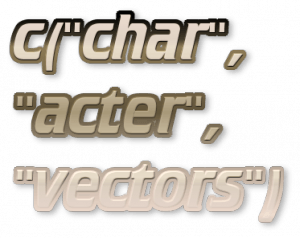Want to share your content on R-bloggers? click here if you have a blog, or here if you don't.In the exercises below we cover the basics of character vectors. Before proceeding, first read section 2.6 of An Introduction to R, and the help pages for the `nchar`, `substr` and `sub` functions.

Answers to the exercises are available here.

Exercise 1
If `x <- “Good Morning! “`, find out the number of characters in X
a. 1
b. 14
c. 13

Exercise 2
Consider the character vector ` x <- c (“Nature’s”, “Best “)`, how many characters are there in x?
a. 12
b. 13
c. 8,5

Exercise 3
If ` x <- c("Nature’s"," At its best ") `, how many characters are there in x?
a. 19
b. 8, 15
c. 8, 9

Exercise 4
If ` fname <- “James“ ` and ` lname <- “Bond”`, write some R code that will produce the output "James Bond".

Exercise 5
If `m <- “Capital of America is Washington” ` then extract the string “Capital of America” from the character vector m.

Exercise 6
Write some R code to replace the first occurrence of the word “failed” with “failure” in the string “Success is not final, failed is not fatal”.

Exercise 7
Consider two character vectors:
`Names <- c("John", "Andrew", "Thomas") ` and
`Designation <- c("Manager", "Project Head", "Marketing Head")`.
Write some R code to obtain the following output.
Names Designation
1 John Manager

Exercise 8
Write some R code that will initialise a character vector with fixed length of 10.

Exercise 9
Write some R code that will generate a vector with the following elements, without using loops.
"aa" "ba" "ca" "da" "ea" "ab" "bb" "cb" "db" "eb" "ac" "bc" "cc" "dc" "ec"
"ad" "bd" "cd" "dd" "ed" "ae" "be" "ce" "de" "ee"

Exercise 10
Let ` df <- data.frame(Date = c("12/12/2000 12:11:10")) `. Write some R code that will convert the given date to character values and gives the following output:
"2000-12-12 12:11:10 GMT"